# Representation with a highest weight vector

(diff) ← Older revision | Latest revision (diff) | Newer revision → (diff)

A linear representation (cf. Representation of a Lie algebra)of a finite-dimensional semi-simple split Lie algebraover a fieldof characteristic zero with a split Cartan subalgebra, having the following properties.

1) In the spaceofthere is a cyclic vector(i.e.is the smallest-invariant subspace containing).

2)for all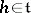, whereis some fixed linear form onwith values in.

3) If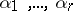is a system of simple roots, defined by a lexicographical order on the setof all roots ofrelative to(cf. Root system), and if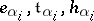are the vectors from the Chevalley basis ofcorresponding to,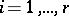, then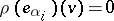for all. Thus,is a weight relative to the restriction ofto(cf. Weight of a representation of a Lie algebra); it is called a highest weight. The spaceis called a cyclic-module with highest weightand generator, andis called a highest weight vector.

There exists for every linear formona unique, up to equivalence, irreducible representation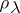ofwith highest weight. The-module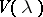determined byis a direct sum of weight subspaces relative to the restriction ofto. Their weights have the formwhere the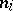are non-negative integers. The weight subspace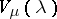of weightis finite-dimensional, spanned overby vectors of the formand for anythe restriction of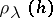tois the operator of scalar multiplication by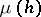. The spaceis one-dimensional; the weightis the only highest weight ofand can be characterized as the unique weight of the-modulesuch that any other weight has the formwhere theare non-negative integers.

A representationis finite-dimensional if and only ifis a dominant linear form on, i.e.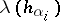is a non-negative integer for. Every irreducible finite-dimensional linear representation ofhas the formfor some dominant linear formon(hence all such representations are classified, up to equivalence, by the dominant linear forms on). The set of all weights of a finite-dimensional representationrelative tois invariant relative to the Weyl group of(regarded as a group of linear transformations of), and if weightsandbelong to one orbit of the Weyl group, then the dimensions of the spacesand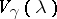are equal. For every weightand every rootthe number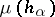is an integer; if, moreover,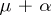is also a weight, then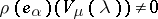(here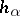is the element incorresponding toand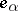is the root vector of).

How to Cite This Entry:
Representation with a highest weight vector. Encyclopedia of Mathematics. URL: http://encyclopediaofmath.org/index.php?title=Representation_with_a_highest_weight_vector&oldid=11726
This article was adapted from an original article by V.L. Popov (originator), which appeared in Encyclopedia of Mathematics - ISBN 1402006098. See original article